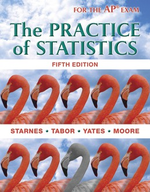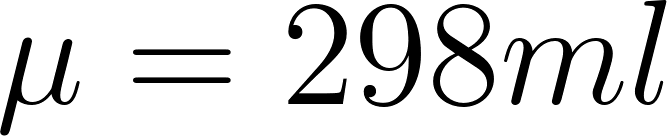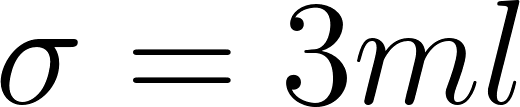×
Get Full Access to The Practice Of Statistics - 5 Edition - Chapter 7.3 - Problem 55
Get Full Access to The Practice Of Statistics - 5 Edition - Chapter 7.3 - Problem 55

×

# What is the probability that a randomly selected coke bottle contains less than 295 ml?ISBN: 9781464108730 488

## Solution for problem 55 Chapter 7.3

The Practice of Statistics | 5th Edition

• Textbook Solutions
• 2901 Step-by-step solutions solved by professors and subject experts
• Get 24/7 help from StudySoup virtual teaching assistantsThe Practice of Statistics | 5th Edition

4 5 1 364 Reviews
18
5
Problem 55

Bottling cola A bottling company uses a filling machine to fill plastic bottles with cola. The bottles are supposed to contain 300 milliliters (ml). In fact, the contents vary according to a Normal distribution with mean $$\mu=298$$ ml and standard deviation $$\sigma=3$$ ml.

(a) What is the probability that a randomly selected bottle contains less than 295 ml ? Show your work.

(b) What is the probability that the mean contents of six randomly selected bottles are less than 295 ml? Show your work.

Step-by-Step Solution:

Step 1 of 3
There should be X amount of cola filled in each of the plastic bottles. It follows a normal distribution.

The mean of the distribution isThe standard deviation isStep 2 of 3

Step 3 of 3

##### ISBN: 9781464108730

The Practice of Statistics was written by and is associated to the ISBN: 9781464108730. This full solution covers the following key subjects: . This expansive textbook survival guide covers 44 chapters, and 1409 solutions. The full step-by-step solution to problem: 55 from chapter: 7.3 was answered by , our top Statistics solution expert on 03/19/18, 03:52PM. This textbook survival guide was created for the textbook: The Practice of Statistics, edition: 5. Since the solution to 55 from 7.3 chapter was answered, more than 453 students have viewed the full step-by-step answer. The answer to “?Bottling cola A bottling company uses a filling machine to fill plastic bottles with cola. The bottles are supposed to contain 300 milliliters (ml). In fact, the contents vary according to a Normal distribution with mean $$\mu=298$$ ml and standard deviation $$\sigma=3$$ ml.(a) What is the probability that a randomly selected bottle contains less than 295 ml ? Show your work.(b) What is the probability that the mean contents of six randomly selected bottles are less than 295 ml? Show your work.” is broken down into a number of easy to follow steps, and 82 words.

## Discover and learn what students are asking

Statistics: Informed Decisions Using Data : Two-Way Analysis of Variance
?To graphically display the role interaction plays in a factorial design, we draw ______.

Unlock Textbook Solution

Enter your email below to unlock your verified solution to:

Bottling cola A bottling company uses a filling machine to fill plastic bottles with